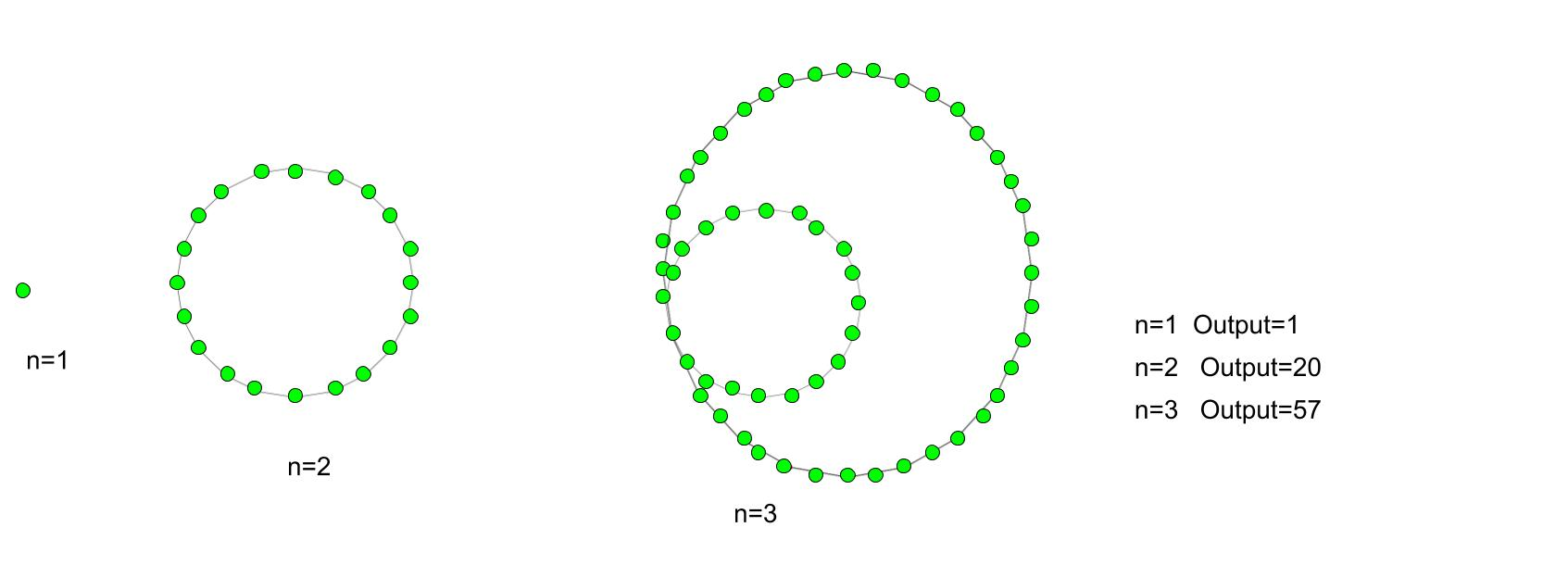# Icosagonal number

Given a number n, the task is to find the nth Icosagonal number.
An Icosagonal number is the 20-gon is a twenty-sided polygon. The number derived from the figurative class. There are different pattern series number in this number. The dots are countable, arrange in a specific way of position and create a diagram. All the dots have a common dots points, all others dots are connected to this points and except this common point the dots connected to their i-th dots with their respective successive layer.

Examples :

Input : 3
Output :57

Input :8
Output :512Formula for nth icosagonal number:## C++

 // C++ program to find  // nth Icosagonal number  #include  using namespace std;     // Function to calculate Icosagonal number  int icosagonal_poly(long int n)  {      // Formula for finding      // nth Icosagonal number      return (18 * n * n - 16 * n) / 2;  }     // Drivers code  int main()  {      long int n = 7;      cout << n << "th Icosagonal number :"                 << icosagonal_poly(n);         return 0;  }

## Java

 // Java program to find  // nth Icosagonal number     import java.io.*;     class GFG {         // Function to calculate Icosagonal number     static int icosagonal_poly(int n)  {      // Formula for finding      // nth Icosagonal number      return (18 * n * n - 16 * n) / 2;  }     // Drivers code             public static void main (String[] args) {            int n = 7;             System.out.print (n + "th Icosagonal number :");      System.out.println(icosagonal_poly(n));      }  }  // This code is contributed by aj_36

## Python 3

 # Python 3 program to find   # nth Icosagonal number     # Function to calculate  # Icosagonal number  def icosagonal_poly(n) :             # Formula for finding      # nth Icosagonal number      return (18 * n * n -              16 * n) // 2    # Driver Code  if __name__ == '__main__' :      n = 7     print(n,"th Icosagonal number : ",                      icosagonal_poly(n))     # This code is contributed m_kit

## C#

 // C# program to find  // nth Icosagonal number  using System;     class GFG  {     // Function to calculate  // Icosagonal number  static int icosagonal_poly(int n)  {      // Formula for finding      // nth Icosagonal number      return (18 * n * n -               16 * n) / 2;  }     // Driver code  static public void Main ()  {         int n = 7;     Console.Write(n + "th Icosagonal " +                          "number :");  Console.WriteLine(icosagonal_poly(n));  }  }     // This code is contributed by ajit

## PHP

 

Output :

7th Icosagonal number :385


Attention reader! Don’t stop learning now. Get hold of all the important DSA concepts with the DSA Self Paced Course at a student-friendly price and become industry ready.

My Personal Notes arrow_drop_upCheck out this Author's contributed articles.

If you like GeeksforGeeks and would like to contribute, you can also write an article using contribute.geeksforgeeks.org or mail your article to contribute@geeksforgeeks.org. See your article appearing on the GeeksforGeeks main page and help other Geeks.

Please Improve this article if you find anything incorrect by clicking on the "Improve Article" button below.

Improved By : jit_t